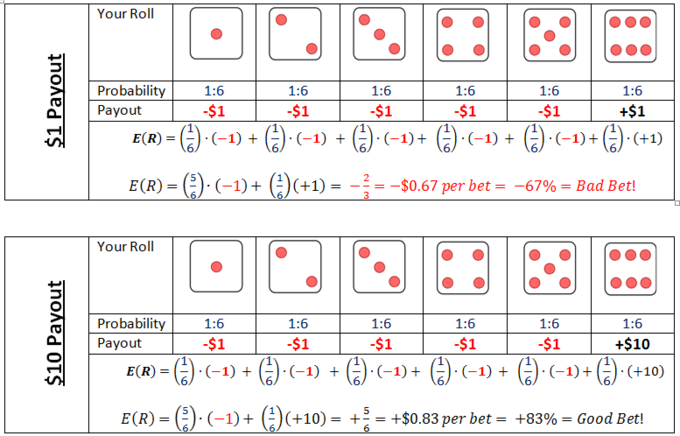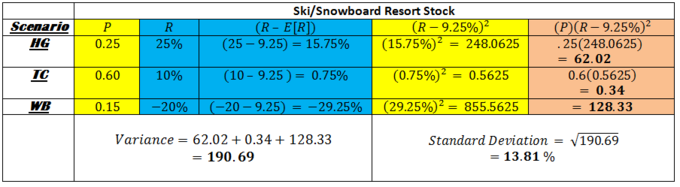## Expected Return

In probability theory, the expected value of a random variable is the weighted average of all possible values.

### Learning Objectives

Calculate the expected return of an investment portfolio

### Key Takeaways

#### Key Points

• In order to make investment decisions, investors often estimate the expected return of a potential investment.
• Expected value is a concept that the helps investors assess the value of a potential investment based on different future outcomes and a probability for each outcome.
• Once you have categories for different scenario ‘s, along with probabilities and returns in each scenario, you then calculate your expected return by multiplying each probability by it’s respective outcome and adding these all together.

#### Key Terms

• expected return: The expected return of a potential investment can be computed by computing the product of the probability of a given event and the return in that case and adding together the products in each discrete scenario.
• expected value: The expected value of a random variable is the weighted average of all possible values that this random variable can take on.

Imagine that your friend offered you a chance to play his game of dice. You have to pay $1 to play and he keeps your money if you roll anything other than a 6. Would you play it? If you answered “it depends”, you are ready to learn about expected value.Care to roll the dice?: Is a bet that doesn’t always pay out, but pays out big when it does a good bet? Is playing the lottery a good bet? Would it be a good bet if tickets were only$0.10 instead of $1.00? If your friend told you he would pay you$1 every time you roll a 6, you would be crazy to play. If your friend told you he would pay you $10 every time you roll a 6, you would be crazy not to play. Expected value is calculated by multiplying the probability that something will happen by the resulting outcome if it happens. In the two cases described above, there are 5 ways to lose and only 1 to win. But in scenario 1, you can expect to lose$0.67 or 67% every time you bet, and in scenario two, you can expect to win $0.83 or 83% with each bet. This is a confusing topic in statistics and finance because on any given roll, there are only two outcomes — win or lose — and neither outcome involves$0.67 or $0.83 increments. But to understand expected value, you have to imagine playing a particular game hundreds of times. If you were to sit at your friend’s apartment and play the dice game 100 times, imagine what your bottom line would be. Even though you lose most of the time you roll in the 2nd scenario, when you win, you win big. After 100 games, you could expect to be up ($0.83 per roll) x (100 rolls) = $83. If you were foolish enough to play the game in the first scenario 100 times you would expect to be down$67 to your friend.

In finance, evaluating your expected return is important, but never as simple as evaluating a game of dice. Imagine that you are going to buy a house a year from today and you have $20,000 saved for that investment. Because you are smart enough to be using a free online text book, you are probably savvy enough to know that you can invest the money for a year and get some return on it. You are considering investing that money into stock of a Ski/Snowboard Mountain in Colorado, so you go talk to your snowboarding friend who lives on your floor of the dorm. He tells you that any given winter could be hella gnarly, totally chillax or wicked bogus, depending on how much it snows. You start doing some research, and you realize that how the stock has performed has everything to do with how much it snows. You create three different categories based on snowfall and label them hella gnarly (HG), totally chillax (TC) and wicked bogus (WB). In recent HG winters, those with over 20 feet of annual snowfall, the stock has averaged a 25% annual return. In recent TC winters, those with between 10 feet and 20 feet of snowdrop throughout the season, the stock has averaged 10%. In WB winters, those with less than 10 feet of total snowdrop, the stock has averaged -20%. Ok, so now what? Should you buy the stock? If you said “it depends,” that’s a good answer. Being as smart as you are, you investigate recent weather patterns and you decide that there is a 25% chance of an HG year, a 60% chance of a TC year and only a 15% chance of a WB winter. You understand that past performance is never a guarantee of future results, but still you are happy with your research and you project an expected rate of return for your$20,000. How will it do?

Based on your research, you realize that the stock has an expected return that is calculated thus:

E[R]= (ProbabilityHG)x(ReturnHG)+(ProbabilityTC)x(ReturnTC)+(ProbabilityWB)x(ReturnWB) = (0.25)(25%) + (0.60)(10%)+(0.15)(-20%) = 6.25% + 6% + -3% = 9.25%

If you were to invest the stock in the ski mountain, year after year, and your research proves accurate, you could expect to receive an average of 9.25% return each year. That is your expected return.

## Variance

Variance is a statistical concept describing the range around expected return within which an investment return can be reasonably expected to fall.

### Learning Objectives

Explain the importance of a stock’s variance and standard deviation

### Key Takeaways

#### Key Points

• Any investment should be made taking time considerations and risk tolerance into account. If there is a specific deadline for when the investment needs to be matured (i.e. to generate retirement income, pay for a down payment on a house, college tuition) then caution is required.
• Three different asset classes — stocks, bonds, money markets — range from aggressive, to moderate, to conservative. An investment that is aggressive typically features a higher expected return, but also a higher variance.
• Variance is calculated by calculating an expected return and summing a weighted average of the squared deviations from the mean return.

#### Key Terms

• variance: In finance, variance is a term used to measure the degree of risk in an investment. It is calculated by finding the average of the squared deviations from the mean rate of return.
• standard deviation: The standard deviation of an investment is obtained by taking the square root of the variance. It has a more straightforward meaning than variance. It tells you that in a given year, you can expect an investment’s return to be one standard deviation above or below the average rate of return.

### Introducing Variance

In probability theory and statistics, the variance is a measure of how far a set of numbers is spread out. It is one of several descriptors of a probability distribution, describing how far the numbers lie from the mean (expected value).

Understanding the concept of variance along with three typical asset classes — money market, bonds, stocks — can help you build a portfolio for any investor. Money market investments are very safe, they almost never go in the red, but they also don’t pay high returns. Stocks are on the opposite end of the spectrum, going back and forth between red and black from year to year frequently, but over longer periods of time they usually pay higher premiums. Bonds are somewhere in the middle. They are safer than a stock, but riskier than a money market and their average returns reflect that.

This table shows how to calculate the variance of an investment outcome.How much do investors want to pay to have to take the good with the bad?: Calculating variance is a 3 step process once expected return has been calculated. Calculate deviations from mean (blue), square the deviations (yellow), multiply the squared deviation by its original probability (orange). Get brownie points by taking the square root of that number and interpret its meaning in the form of a sentence.

You may not need to calculate variance yourself, but you should still notice how we got it. In the figure, we started with three scenarios and a probability (P) and return (R) associated with each. We did some math and ended up using the two blue columns to get the yellow one. Then we multiplied the two yellow columns to get the orange one. We get the variance from adding up the numbers in the orange column. Of the three numbers, we add (62, 0.34, and 128). Two are very big and one is very small. The small number comes from the TC scenario where the stock returns 10%, which is very close to our expectation of 9.25%. The bigger numbers come from winters that are extreme — when the stock performs way above 9.25% (HG) or way below it (WB). The standard deviation can be read as a percentage. It means that, even though we can expect an average of 9.25% return on our stock over the course of 50 years, if we take any given year out and look at its performance, it is likely be somewhere within 13.81% above or below that figure.

### Variance in Relation to Expected Return

In the discussion of expected return, we concluded that, based on your research, you can expect the Ski/Snowboard Resort in Colorado to have an expected return of 9.25% based on three distinct weather outcomes. However, if you invest your $20,000 in that company and expect to have$21,850 after a year, you must remember that this isn’t a dice game that you can play over and over again. There will only be one result in this case and at the end of it, you have to make a down payment on a house. Is this a good investment idea?

What if your bid for a house won’t be accepted unless you can put at least $20,000 down? There is an 85% chance that the winter is either hella gnarly (HG) or totally chillax (TC), and in either of those cases you will still have over$20,000 to make a down payment. There is also a 15% chance that the year ends up being wicked bogus (WB), and if that is the case you will lose 20% or $4,000 of your initial investment. Now you have$16,000 and all of a sudden you are thinking tree fort. Let’s compare that investment to a CD at a bank that pays 3.25% no matter how much snow falls this winter. You can have an investment that is federally insured to pay you $20,650 one year from today and you can be assured to have enough to make a down payment on your house. If you can let that$20,000 investment sit for 10 years before you need it, there is a much better chance that you will end up in the black (experiencing a profit) than in the red (experiencing a loss). A 30 year old with a 401K can be much more aggressive in his portfolio than a 65 year old who will be retiring in one year can. Every portfolio should be modeled with time-frame and risk tolerance considerations. It would be just as foolish for the 65 year old to be investing in aggressive stocks as it would for the 30 year old to buy conservative CD’s in his retirement account.